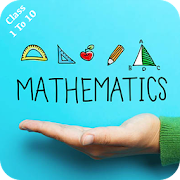# Mathematics For Class 1 To 10 Students - Lumos Educational App Store3.91
Price -FREE
\$0

#### DESCRIPTION:

Best app for Mathematics For Class 1 To 10 Students. Fun Math Tricks: 1. Addition 2. Subtraction 3. Multiplication table 4. Division 5. Multiply two digit number by 11 6. Square numbers ending in 5 7. Multiply by 5 8. Multiply by 9 9. Multiply by 4 10. Dividing by 5 11. Subtracting from 1000 12. Tough multiplication 13. Power of two 14. Adding numbers close to hundreds 15. Subtracting numbers close to hundreds 16. Multiply numbers between 11 and 19 17. Square numbers between 11 and 99 18. Multiply two digit numbers having same tens digit and ones digits add up to ten 19

#### OVERVIEW:

Mathematics For Class 1 To 10 Students is a free educational mobile app By .It helps students in grades HS practice the following standards HSN.CN.B.5.

This page not only allows students and teachers download Mathematics For Class 1 To 10 Students but also find engaging Sample Questions, Videos, Pins, Worksheets, Books related to the following topics.

1. HSN.CN.B.5 : Represent addition, subtraction, multiplication, and conjugation of complex numbers geometrically on the complex plane; use properties of this representation for computation. For example, (-1 + √3 i)3 = 8 because (-1 + √3 i) has modulus 2 and argument 120°..

HS

#### STANDARDS:

HSN.CN.B.5

Developer:

Software Version: 1.2

Category: Education

### RELATED APPSEdSearch WebSearch